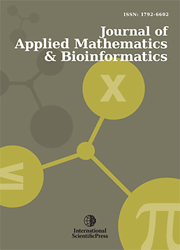# Journal of Applied Mathematics & Bioinformatics

#### Rating the Security Strength of Cryptographic Algorithms

•[ Download ]
• Abstract

In this study, we propose a method in order to estimate the strength of a cryptographic algorithm. The method combines the evaluation of the cryptographic key length and the evaluation of the success rate of the randomness tests in the algorithm output samples. In the first step, the algorithm is classified into one of four general categories, according to its key size, taking into account the current computer power which a cryptanalyst can use for exhaustive key search. In the second step, we examine the success rate of the tests on the output samples. For this, the maximum accepted number of the rejected samples is calculated, taking as parameters the total number of samples (which depends from the selected sampling error) and the desired significance level and confidence interval for the success rate of the tests. If the rejected samples do not exceed the maximum number, the algorithm is considered as “random” and it is rated in the initial strength category due to its key size. If the rejected samples exceed the maximum number, the algorithm is submitted to further tests under certain conditions.

Keywords: Cryptography, Data encryption, Communication security, Computer security, Data security, Information security.ISSN: 1792-6939 (Online)
1792-6602 (Print)